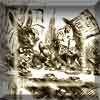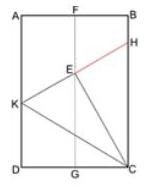#### You may also like### A Problem of Time

Consider a watch face which has identical hands and identical marks for the hours. It is opposite to a mirror. When is the time as read direct and in the mirror exactly the same between 6 and 7?The points P, Q, R and S are the midpoints of the edges of a non-convex quadrilateral.What do you notice about the quadrilateral PQRS and its area?

# Making Sixty

##### Age 14 to 16 Challenge Level:

Congratulations to all those who submitted solutions. Billy from Sedgehill School drew a diagram showing sides and angles he knew must be equal, which helped him to spot 30 and 60 degree angles. Patrick from Woodbridge School went a little further and made a good argument using ideas about congruent triangles. Jack's (Fast Forward) solution was also very clear and used similar ideas to Patrick's; I have combined their solutions below.I know that KEC is a right angle because it is the corner of the paper, so as KH is a straight line, CEH is also a right angle. The line KE = EH; I know this because E lies on FG which divides the sheet in two. EC is common to both triangles, so triangles KEC and CEH are congruent (Side-Angle-Side).

I know that triangle KDC is also congruent to KEC because we folded corner D up to E to create the two triangles.

As the three triangles are congruent, I know that angles DCK, KCE and ECH are all equal, so as DCH is a right angle, each angle must be $90 \div 3$ which is $30$ degrees.

Angles in a triangle add up to $180$ degrees, so angle CKE and CHE are $180-90-30=60$ degrees, so triangle CKH is equilateral.